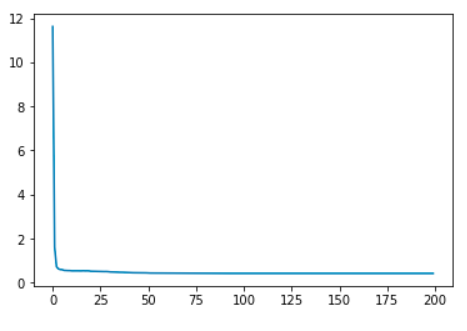# Loss flattens out

I have worked on a couple of projects now where I have constructed a neural net for binary classification and something like this has happened. This leads me to believe it’s something to do with how I am programming it rather than the data itself.

I have tried all kinds of things, even posted here before but I’m not sure why. I did not recycle my own code or anything so I don’t know how this problem persists across projects.

The model is simple:

``````# A simple binary classification model

class BinaryClassifier(nn.Module):

def __init__(self, input_size, hidden_size, num_classes=1):
super().__init__()

self.input_size = input_size
self.num_classes = num_classes

self.linear1 = nn.Linear(input_size, hidden_size)
self.linear2 = nn.Linear(hidden_size, num_classes)

self.relu = nn.ReLU()

def forward(self, x):
x = self.linear1(x)
x = self.relu(x)
x = self.linear2(x)
return x
``````

I have set num_classes as 1 in order to get a probability that the label is 1. I wasn’t sure how else to structure this binary classification.

I use BCEWithLogitsLoss, Adam with a StepLR scheduler

``````model = BinaryClassifier(X_tensor.shape, 512)
criterion = nn.BCEWithLogitsLoss()
scheduler = torch.optim.lr_scheduler.StepLR(optimizer, step_size=10, gamma=0.5)
``````

It’s worth mentioning here I only added the scheduler to fix the problem and it did improve the loss but not the flattening out effect.

This is how I train the model:

``````def sub_train_(model, dataloader):
model.train()
losses = list()
for idx, (X, y) in enumerate(dataloader):

out = model(X)
loss = criterion(out, y.unsqueeze(1))

loss.backward()
optimizer.step()
losses.append(loss.item())
return np.mean(losses), model
``````
``````def train(model, trainloader, testloader, scheduler, n_epochs):
best_model = model
best_loss = math.inf
ts = time.time()

losses = list()

for epoch in range(n_epochs):
scheduler.step()
losses.append(train_loss)

if train_loss < best_loss:
best_loss = train_loss
best_model = model

print('Epoch: {}, train_loss: {}, test_loss: {}'.format(
epoch, train_loss, test_loss
))
te = time.time()

fig, ax = plt.subplots()
ax.plot(range(n_epochs), losses)
plt.show()

mins = int((te-ts) / 60)
secs = int((te-ts) % 60)

print('Training completed in {} minutes, {} seconds.'.format(mins, secs))
return losses, best_model
``````

And every time it yields a loss plot like this:What am I doing wrong here? How can I avoid this happening?

Thank you in advance to anyone who helps me out with this!

First of all, you probably want two classes. Solving a problem with one class is impossible, meaning that you always have at least two classes. In your cases: an element has a class (class 1), or it hasn’t (class 2). So num classes should be two. Make sure that your labels are constructed correctly.

Also, loss flattening means that the network is learning, which is a good thing. However, because you are not running on a test set, it is very likely that your model just overfits. You can Google ‘prevent overfitting’ and you’ll find solutions such as dropout.

Hi @BramVanroy Thanks for your response!

Yes my labels could be structured better. So with BCEWithLogitsLoss, what is the standard practice for how to structure the labels? At the moment I simply have a label column with a 1 or a 0, where 0 is one class and 1 is the other. What is the better way to do it?

I am running it on a test set as well. Ah I hadn’t put the function in the post but I do make a call to it in my train function. However that doesn’t have any impact on the training so what can I do to make my loss decrease even further?

From what I read, we don’t have to use a one-hot vector for 2 classes when using BCEWithLogitLoss. We just need to give a single label column as you have done. So, I think the num_classes should still be 1. Like in https://towardsdatascience.com/pytorch-tabular-binary-classification-a0368da5bb89